# Stable and unstable theories

(diff) ← Older revision | Latest revision (diff) | Newer revision → (diff)
A branch of model theory dealing with the stability of elementary theories (cf. Elementary theory). Letbe a complete theory of the first order, of signature (language), letbe a model ofand let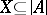. The signatureis obtained fromby adding isolated element symbols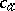for all. The systemhas signature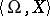and is an enrichment (simple expansion) of the model, in whichis interpreted asfor all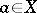. The theoryis the totality of formulas of signaturethat are true in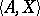. A set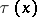of formulas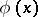in the languagewith one free variableis a type ofif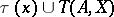is satisfiable.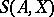is the collection of all maximal types of. The theoryis said to be stable at cardinalityif for any modelofand anyof cardinality not exceeding, the cardinality ofalso does not exceed. A theory is called stable if it is stable at even one infinite cardinality.
Let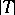denote the cardinality of the set of formulas of signature. Ifis stable, then it is stable at all cardinalities that satisfy the equality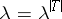. Ifis stable, then there exist a modelofand an infinite set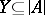such that for any formula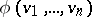of signatureand for any two sequences,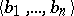of different elements of, the truth of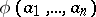inis equivalent to the truth of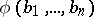in; the setis then called the set of indistinguishable elements in. A characteristic property of unstable theories is the existence of a set which has somehow opposite properties. Namely, the instability of a theoryis equivalent to the existence of a formulaof signature, of a modelofand of a sequence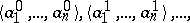of tuples of elements of, such that the truth of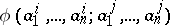inis equivalent to the inequality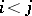. For this reason, complete extensions of the theory of totally ordered sets with infinite models, as well as the theory of any infinite Boolean algebra, are unstable. In particular, the theory of natural numbers with addition and the theory of the field of real numbers are unstable. If a theoryis unstable, then the number of isomorphism types of models ofat every uncountable cardinal number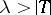is equal to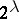. A theorythat is categorical at an uncountable cardinal number(cf. Categoricity in cardinality) is therefore stable. There do exist stable theories, however, that are not categorical at any infinite cardinality. Such an example is the theorywhose signature consists of a one-place predicate and a countable set of isolated elements. The axioms of this theory state that a predicate is true on the isolated elements, divides every model of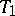into two infinite sets, and that the isolated elements are not equal to each other.
Theories of finite or countable signature that are stable at a countable cardinality are also said to be totally transcendental. Every totally transcendental theory is stable at all infinite cardinalities. Every categorical theory of finite or countable signature at an uncountable cardinality is totally transcendental. The theoryabove is totally transcendental. Totally transcendental theories can also be characterized in other terms. Letbe a complete theory of finite or countable signatureand letbe an infinite model of. A formula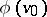of signatureis given the rank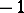if it is false on all elements of the model, and the rank(is an ordinal number) if it does not have any rank lower than; however, for every elementary extensionof the system, and for every formula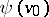of signature, one of the formulas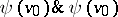or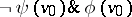is given a rank less than. A theoryis totally transcendental if and only if for every modelof, each formulaof signatureis given a certain rank.